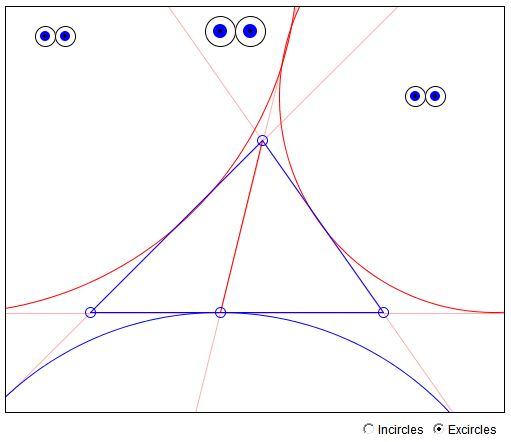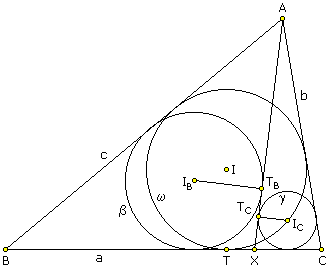Property of Points Where In- and Excircles Touch a TriangleWhat Is It About? A Mathematical Droodle

If you are reading this, your browser is not set to run Java applets. Try IE11 or Safari and declare the site https:///www.cut-the-knot.org as trusted in the Java setup.What if applet does not run?

ExplanationIf one drags the intermediate point on the base of the triangle sufficiently slow, the applet may suggest the following

Theorem 1

Let the incircle of ΔABC touch the side BC at point X. Then incircles of triangles ABX and ACX touch the line AX at the same point P.The theorem appears in [Honsberger, exercise 2, chapter 1] (see also The Second Canadian Open Mathematics Challenge, problem 6). It was observed by Vladimir Zajic that the theorem admits a generalization whose proof is only slightly different from the one that appears in [Honsberger].

Theorem 1'

Let point X belong to the base BC of triangle ABC. Let T be the point where the incircle of triangle ABC touches BC. Construct incircles of triangle ABX and ACX, and assume that they touch AX at points TB and TC, respectively. Then TX = TBTC.Note that Theorem 1 follows from Theorem 1' when T = X.

Proof of Theorem 1'

The proof refers to the above configuration, where the inradius of ΔABX is greater than the inradius of ΔACX. The other case is completely analogous.

Denote the sides of ΔABC in the usual way: a = BC, b = CA, and c = AB. Let s, sB, and sC be semiperimeters of the triangles ABC, ABX, and ACX, respectively. By simple algebra, the distance from a triangle vertex to the tangency point with the incircle equals to the difference between the semiperimeter and the opposite side of the triangle. In ΔABC, we have

BX = BT + TX = s - b + TX
CX = CT - TX = s - c - TX.

Similarly, in triangles ABX and ACX, we have

XTB = sB - c
XTC = sC - b.

Therefore

 TBTC = XTB - XTC = (sB - c) - (sC - b) = sB - sC + b - c

Since by definition

2sB = c + AX + BX
2sC = b + AX + CX

it follows that

 2(sB - sC) = c - b + BX - CX = c - b + (s - b + TX) - (s - c - TX) = 2(c - b + TX)

In other words,

sB - sC = c - b + TX

Therefore,

 TBTC = sB - sC + b - c = c - b + TX + b - c = TX.

Q.E.D.

Remark

Fix the lines through A and X (l) and that through B and C (k). Then X is also fixed. Now choose two circles b and g inscribed into angles AXB and AXC. Let A slide over l, and consider triangles ABC formed by the tangents AB and AC from A to the two circles b and g. If A is sufficiently removed from k so that the resulting configuration is the one depicted above, then the incenters of triangles ABC all project into the same point on k.

Remark 2

Theorem 1 is a direct consequence of a theorem about incircles in a quadrilateral: Together with its sides, the diagonals of a quadrilateral form two pairs of triangles: ABC, ADC and BCD, BAD. One pair shares the side AC, the other the side BD. In each of the four triangles draw its incircle. The applet illustrates the following theorem: the two incircles in one pair touch each other iff the same is true of the two incircles in the other pair. (Two incircles of adjacent triangles may only touch each other if the points where they touch the common side coincide.)

As we know, the condition that two circles in a pair are tangent is equivalent to the quadrilateral being inscriptible. So inscribe a circle into the quadrilateral. Move two adjacent tangency points towards each other until they coalesce. One of the vertices will then move into that double tangency point, and the quadrilateral will degenerate into a triangle. One of the diagonals will join the double tangency point with the opposite vertex and the whole configuration will becaome that of Theorem 1.

References

1. R. Honsberger, Episodes in Nineteenth and Twentieth Century Euclidean Geometry, MAA, 1995.•